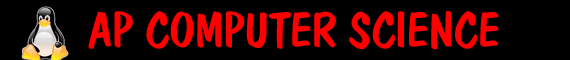Assignments:   I. Modify the Account class so that it has these new methods: add a new variable for interestRate add methods getInterestRate and setInterestRate add method addInterest that will not return anything but will add the interest to the balance. Interest is calculated as the rate times the balance. Ie. if interestRate was .05 and the balance was 300, than when addInterest was called, the new balance would be 315 (300 + .05*300) II. Create a new class for a Die (singular of Dice). private int numOfSides private int face public Die () public Die (numOfSides) public int roll() public String toString() public int getFace() III. Create a class for Die called TwoDice. private Die die1 private Die die2 public TwoDice() //initialize 2 dice to have 6 sides public TwoDice(int numOfSides) //initialize 2 die w/ numOfSides public TwoDice (int numOfSides1, int numOfSides2) public int roll () //rolls the dice and returns total public int getTotal() //returns the total public boolean isDoubles() //returns true or false if doubles public String toString() //print each die   Sample code to test your DiceTester - just put in as a new class in eclipse. ``` public class DiceTester { /** * @param args */ public static void main(String[] args) { Die testDie=new Die(); testDie.roll(); System.out.println("Test Die is showing " + testDie.getFace()); System.out.println(testDie.toString()); TwoDice testDice=new TwoDice(10,14); testDice.roll(); System.out.println("Test Die is showing " + testDice.getTotal()); System.out.println(testDice.toString()); if (testDice.isDoubles()) System.out.println("You rolled doubles"); else System.out.println("You did not roll doubles"); } } ```   IV. Create a class called DiceGame that will play a stupid betting game. In it the person starts out with \$500 and they are given the opportunity to 1)bet 2)quit If they bet, ask them how much money they would like to bet? If they quit, display how much money they have now. After they have placed a bet, the computer will roll first, showing what it has rolled. It will then ask you to hit enter to roll, then roll and show what you got. The winner is the one with: high double beat low doubles doubles beat non doubles then higher roll beats lower roll It will compute the new total and the game begins again. To find out how much they want to bet, you might say int bet = Integer.parseInt(readString());